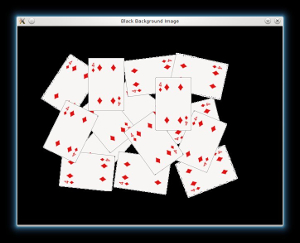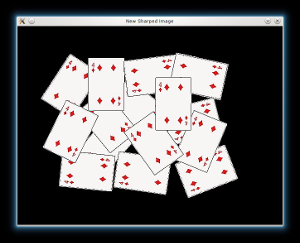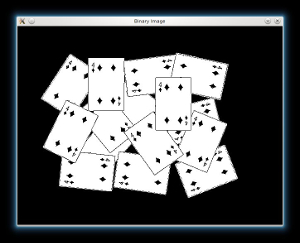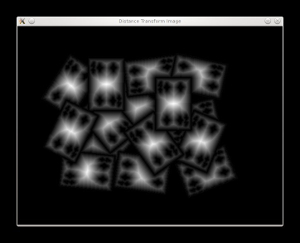Posted on

## 函數介紹

`cv2.floodFill()` 函數可以用來對圖像進行泛洪填充。泛洪填充是指將圖像中指定的像素點及其相連的像素點填充成指定的顏色。它通常用於圖像的背景去除、圖像分割等應用中。常用的場景如下:

1. 圖像分割：可以使用泛洪填充來將圖像分割成不同的區域，例如可以從圖像中自動分離出前景和背景。
2. 圖像去噪：可以使用泛洪填充來去除圖像中的噪聲，例如在二值化圖像中可以填充噪點附近的像素，使其與周圍的像素保持一致。
3. 圖像修復：可以使用泛洪填充來修復圖像中的缺陷，例如在圖像中填充缺陷周圍的像素，使其與周圍的像素保持一致。
4. 圖像標記：可以使用泛洪填充來對圖像進行標記，例如對圖像中的區域進行標記，或者在圖像中添加文字等。

## 參數介紹

`cv2.floodFill()` 函數的常用參數如下：

```cv2.floodFill(image, mask, seedPoint, newVal[, rect[, loDiff[, upDiff[, flags]]]]) -> retval, image, mask, rect
```
• `image`：要填充的圖像，必須為8位、單通道或三通道影像。如果是三通道影像，則只有當 `flags` 參數中包含 `cv2.FLOODFILL_FIXED_RANGE` 時，填充才會基於每個像素的三通道值。
• `mask`：用於指定填充區域的填充標記，必須為單通道、8位或32位浮點數影像，大小應比 `image` 多2個像素。如果填充標記中對應位置的值為0，則該像素將不會被填充。如果該參數為 `None`，則會自動創建一個和 `image` 大小相同的標記。
• `seedPoint`：種子點的位置，是一個二元數組 `(x, y)`
• `newVal`：填充的新值，可以是一個標量或一個三元數組 `(B, G, R)`
• `rect`：可選的輸出參數，用於返回填充區域的最小矩形。
• `loDiff`：可選的最小差值，如果當前像素和種子點之間的差值小於 `loDiff`，則這個像素將被填充。默認值為0。
• `upDiff`：可選的最大差值，如果當前像素和種子點之間的差值大於 `upDiff`，則這個像素不會被填充。默認值為0。
• `flags`：可選的填充標誌，可以是以下幾種取值之一或者它們的組合：
• `cv2.FLOODFILL_FIXED_RANGE`：基於每個像素的三通道值來填充，默認基於灰度值。
• `cv2.FLOODFILL_MASK_ONLY`：僅修改填充標記，不修改圖像。
• `cv2.FLOODFILL_MULTISCALE`：使用多個尺度進行填充。
• `cv2.FLOODFILL_POINT`：表示 `seedPoint` 參數為像素的坐標，而不是像素值。

## 使用範例

```import cv2
import numpy as np

# 讀入圖像，轉為灰度
gray = cv2.cvtColor(img, cv2.COLOR_BGR2GRAY)

# 找到種子點
seed_point = (100, 100)

# 設置填充顏色和填充標記
fill_color = (0, 0, 255)

# 泛洪填充

# 顯示圖像
cv2.imshow('image', img)
cv2.waitKey(0)
cv2.destroyAllWindows()

```
Posted on

## 取得輪廓的矩形邊界框

`cv2.boundingRect()` 函數可以用來計算一個輪廓的矩形邊界框（bounding box），即最小矩形框，這個矩形框可以完全包圍輪廓的所有點。這個函數的返回值是一個元組 `(x,y,w,h)`，其中 `(x,y)` 是矩形框左上角的座標，`w``h` 是矩形框的寬度和高度。

```import cv2

# 讀入圖像，轉為灰度
gray = cv2.cvtColor(img, cv2.COLOR_BGR2GRAY)

# 二值化，尋找輪廓
_, thresh = cv2.threshold(gray, 0, 255, cv2.THRESH_BINARY + cv2.THRESH_OTSU)
contours, _ = cv2.findContours(thresh, cv2.RETR_EXTERNAL, cv2.CHAIN_APPROX_NONE)

# 畫出輪廓
cv2.drawContours(img, contours, -1, (0, 255, 0), 2)

# 尋找最小矩形框
x, y, w, h = cv2.boundingRect(contours)

# 畫出矩形框
cv2.rectangle(img, (x, y), (x+w, y+h), (0, 0, 255), 2)

# 顯示圖像
cv2.imshow('image', img)
cv2.waitKey(0)
cv2.destroyAllWindows()

```

## 最小擬合矩形

`cv2.minAreaRect()` 可計算最小擬合矩形，這個函數會將給定的輪廓點集擬合成一個矩形，這個矩形具有最小面積，可以包圍住所有的輪廓點。

```import cv2

# 讀入圖像，轉為灰度
gray = cv2.cvtColor(img, cv2.COLOR_BGR2GRAY)

# 二值化，尋找輪廓
_, thresh = cv2.threshold(gray, 0, 255, cv2.THRESH_BINARY + cv2.THRESH_OTSU)
contours, _ = cv2.findContours(thresh, cv2.RETR_EXTERNAL, cv2.CHAIN_APPROX_NONE)

# 畫出輪廓
cv2.drawContours(img, contours, -1, (0, 255, 0), 2)

# 尋找最小擬合矩形
rect = cv2.minAreaRect(contours)
box = cv2.boxPoints(rect)
box = np.int0(box)

# 畫出矩形
cv2.drawContours(img, [box], 0, (0, 0, 255), 2)

# 顯示圖像
cv2.imshow('image', img)
cv2.waitKey(0)
cv2.destroyAllWindows()

```

## 取得最小包圍橢圓

```import cv2

# 讀入圖像，轉為灰度
gray = cv2.cvtColor(img, cv2.COLOR_BGR2GRAY)

# 二值化，尋找輪廓
_, thresh = cv2.threshold(gray, 0, 255, cv2.THRESH_BINARY + cv2.THRESH_OTSU)
contours, _ = cv2.findContours(thresh, cv2.RETR_EXTERNAL, cv2.CHAIN_APPROX_NONE)

# 畫出輪廓
cv2.drawContours(img, contours, -1, (0, 255, 0), 2)

# 尋找最小包圍橢圓
ellipse = cv2.fitEllipse(contours)
cv2.ellipse(img, ellipse, (0, 0, 255), 2)

# 尋找最小面積包圍橢圓
ellipse = cv2.minEnclosingEllipse(contours)
cv2.ellipse(img, (int(ellipse), int(ellipse)),
(int(ellipse / 2), int(ellipse / 2)),
ellipse, 0, 360, (255, 0, 0), 2)

# 顯示圖像
cv2.imshow('image', img)
cv2.waitKey(0)
cv2.destroyAllWindows()
```

## 最佳擬合橢圓

`cv2.fitEllipse()` 函數找到的是能夠最好擬合給定點集的橢圓，並不一定能夠包圍住所有點。

```import cv2

# 讀入圖像，轉為灰度
gray = cv2.cvtColor(img, cv2.COLOR_BGR2GRAY)

# 二值化，尋找輪廓
_, thresh = cv2.threshold(gray, 0, 255, cv2.THRESH_BINARY + cv2.THRESH_OTSU)
contours, _ = cv2.findContours(thresh, cv2.RETR_EXTERNAL, cv2.CHAIN_APPROX_NONE)

# 畫出輪廓
cv2.drawContours(img, contours, -1, (0, 255, 0), 2)

# 尋找最小包圍橢圓
ellipse = cv2.fitEllipse(contours)
cv2.ellipse(img, ellipse, (0, 0, 255), 2)

# 顯示圖像
cv2.imshow('image', img)
cv2.waitKey(0)
cv2.destroyAllWindows()

```

## 最小包圍圓

```import cv2
import numpy as np

# 讀入圖像，轉為灰度
gray = cv2.cvtColor(img, cv2.COLOR_BGR2GRAY)

# 二值化，尋找輪廓
_, thresh = cv2.threshold(gray, 0, 255, cv2.THRESH_BINARY + cv2.THRESH_OTSU)
contours, _ = cv2.findContours(thresh, cv2.RETR_EXTERNAL, cv2.CHAIN_APPROX_NONE)

# 畫出輪廓
cv2.drawContours(img, contours, -1, (0, 255, 0), 2)

# 尋找最小外接圓
center = (int(x), int(y))

# 畫出圓形
cv2.circle(img, center, radius, (0, 0, 255), 2)

# 顯示圖像
cv2.imshow('image', img)
cv2.waitKey(0)
cv2.destroyAllWindows()

```

## 最適擬合直線

```import cv2
import numpy as np

# 讀入圖像，轉為灰度
gray = cv2.cvtColor(img, cv2.COLOR_BGR2GRAY)

# 二值化，尋找輪廓
_, thresh = cv2.threshold(gray, 0, 255, cv2.THRESH_BINARY + cv2.THRESH_OTSU)
contours, _ = cv2.findContours(thresh, cv2.RETR_EXTERNAL, cv2.CHAIN_APPROX_NONE)

# 畫出輪廓
cv2.drawContours(img, contours, -1, (0, 255, 0), 2)

# 尋找最適擬和直線
rows, cols = img.shape[:2]
[vx, vy, x, y] = cv2.fitLine(contours, cv2.DIST_L2, 0, 0.01, 0.01)
lefty = int((-x*vy/vx) + y)
righty = int(((cols-x)*vy/vx)+y)

# 畫出直線
cv2.line(img, (cols-1, righty), (0, lefty), (0, 0, 255), 2)

# 顯示圖像
cv2.imshow('image', img)
cv2.waitKey(0)
cv2.destroyAllWindows()

```
Posted on

## 用OPENCV繪製垂直線

```import cv2
import numpy as np

# 創建一個空的圖像，並設置其大小為 100x100 像素，並設置其通道數為 3 (RGB)
img = np.zeros((100, 100, 3), np.uint8)

# 定義點的座標
point1 = (10, 20)
point2 = (50, 30)

# 計算兩個點的中心點座標
center_point = ((point1 + point2) // 2, (point1 + point2) // 2)

# 繪製出兩個點以及中心點
cv2.circle(img, point1, 2, (0, 0, 255), -1)
cv2.circle(img, point2, 2, (0, 0, 255), -1)
cv2.circle(img, center_point, 2, (0, 255, 0), -1)

# 計算與這兩點相連的直線垂直的線的端點座標
if point1 == point2:
# 如果這兩個點的 x 座標相等，則直接在中心點上下各畫一個點，這兩個點就是線的端點
line_point1 = (center_point, 0)
line_point2 = (center_point, 100)
else:
# 否則，計算這兩個點之間的斜率
k = (point2 - point1) / (point2 - point1)
# 計算垂直於這條線的斜率
vk = -1 / k
# 計算線的端點座標
line_point1 = (center_point - 50, int(center_point - vk * 50))
line_point2 = (center_point + 50, int(center_point + vk * 50))

# 繪製出垂直線
cv2.line(img, line_point1, line_point2, (255, 0, 0), 1)

# 顯示圖像
cv2.imshow("image", img)
cv2.waitKey(0)
cv2.destroyAllWindows()
```
Posted on

## 取得輪廓的中心點

```import cv2

# 讀取圖像，並將其轉換為灰度圖像
gray = cv2.cvtColor(img, cv2.COLOR_BGR2GRAY)

# 進行閾值處理，以便更好地找到輪廓
ret, thresh = cv2.threshold(gray, 127, 255, 0)

# 查找輪廓
contours, hierarchy = cv2.findContours(thresh, cv2.RETR_TREE, cv2.CHAIN_APPROX_SIMPLE)

# 繪製輪廓，以便在圖像上進行視覺化
cv2.drawContours(img, contours, -1, (0, 255, 0), 3)

# 計算輪廓的中心點
if len(contours) > 0:
# 取最大的輪廓
c = max(contours, key=cv2.contourArea)
# 計算輪廓的矩
M = cv2.moments(c)
# 計算中心點座標
center_x = int(M["m10"] / M["m00"])
center_y = int(M["m01"] / M["m00"])
# 繪製中心點，以便在圖像上進行視覺化
cv2.circle(img, (center_x, center_y), 5, (255, 0, 0), -1)

# 顯示圖像
cv2.imshow("image", img)
cv2.waitKey(0)
cv2.destroyAllWindows()
```
Posted on

## 如何分割黏在一起的撲克牌

### 範例程式碼

```from __future__ import print_function
import cv2 as cv
import numpy as np
import argparse
import random as rng
rng.seed(12345)
parser = argparse.ArgumentParser(description='Code for Image Segmentation with Distance Transform and Watershed Algorithm.\
Sample code showing how to segment overlapping objects using Laplacian filtering, \
in addition to Watershed and Distance Transformation')
parser.add_argument('--input', help='Path to input image.', default='cards.png')
args = parser.parse_args()
if src is None:
print('Could not open or find the image:', args.input)
exit(0)
# Show source image
cv.imshow('Source Image', src)
src[np.all(src == 255, axis=2)] = 0
# Show output image
cv.imshow('Black Background Image', src)
kernel = np.array([[1, 1, 1], [1, -8, 1], [1, 1, 1]], dtype=np.float32)
# do the laplacian filtering as it is
# well, we need to convert everything in something more deeper then CV_8U
# because the kernel has some negative values,
# and we can expect in general to have a Laplacian image with negative values
# BUT a 8bits unsigned int (the one we are working with) can contain values from 0 to 255
# so the possible negative number will be truncated
imgLaplacian = cv.filter2D(src, cv.CV_32F, kernel)
sharp = np.float32(src)
imgResult = sharp - imgLaplacian
# convert back to 8bits gray scale
imgResult = np.clip(imgResult, 0, 255)
imgResult = imgResult.astype('uint8')
imgLaplacian = np.clip(imgLaplacian, 0, 255)
imgLaplacian = np.uint8(imgLaplacian)
#cv.imshow('Laplace Filtered Image', imgLaplacian)
cv.imshow('New Sharped Image', imgResult)
bw = cv.cvtColor(imgResult, cv.COLOR_BGR2GRAY)
_, bw = cv.threshold(bw, 40, 255, cv.THRESH_BINARY | cv.THRESH_OTSU)
cv.imshow('Binary Image', bw)
dist = cv.distanceTransform(bw, cv.DIST_L2, 3)
# Normalize the distance image for range = {0.0, 1.0}
# so we can visualize and threshold it
cv.normalize(dist, dist, 0, 1.0, cv.NORM_MINMAX)
cv.imshow('Distance Transform Image', dist)
_, dist = cv.threshold(dist, 0.4, 1.0, cv.THRESH_BINARY)
# Dilate a bit the dist image
kernel1 = np.ones((3,3), dtype=np.uint8)
dist = cv.dilate(dist, kernel1)
cv.imshow('Peaks', dist)
dist_8u = dist.astype('uint8')
# Find total markers
_, contours, _ = cv.findContours(dist_8u, cv.RETR_EXTERNAL, cv.CHAIN_APPROX_SIMPLE)
# Create the marker image for the watershed algorithm
markers = np.zeros(dist.shape, dtype=np.int32)
# Draw the foreground markers
for i in range(len(contours)):
cv.drawContours(markers, contours, i, (i+1), -1)
# Draw the background marker
cv.circle(markers, (5,5), 3, (255,255,255), -1)
markers_8u = (markers * 10).astype('uint8')
cv.imshow('Markers', markers_8u)
cv.watershed(imgResult, markers)
#mark = np.zeros(markers.shape, dtype=np.uint8)
mark = markers.astype('uint8')
mark = cv.bitwise_not(mark)
# uncomment this if you want to see how the mark
# image looks like at that point
#cv.imshow('Markers_v2', mark)
# Generate random colors
colors = []
for contour in contours:
colors.append((rng.randint(0,256), rng.randint(0,256), rng.randint(0,256)))
# Create the result image
dst = np.zeros((markers.shape, markers.shape, 3), dtype=np.uint8)
# Fill labeled objects with random colors
for i in range(markers.shape):
for j in range(markers.shape):
index = markers[i,j]
if index &amp;gt; 0 and index &amp;lt;= len(contours):
dst[i,j,:] = colors[index-1]
# Visualize the final image
cv.imshow('Final Result', dst)
cv.waitKey()```

### distanceTransform

distanceTransform函數是OpenCV中的一個函數，用於計算圖像中每個非零點到最近背景像素的距離。distanceTransform函數的第二個Mat矩陣參數dst保存了每個點與最近的零點的距離信息，圖像上越亮的點，代表了離零點的距離越遠。在這篇文章中，作者通過一個實例演示了如何使用distanceTransform函數對圖像進行分割。

### 處理的過程圖片Posted on

## 拆分相黏的正方形

### 原始圖片### 解決問題的思考方向

```from scipy.ndimage import label
import cv2
import numpy
def segment_on_dt(img):
dt = cv2.distanceTransform(img, cv2.DIST_L2, 3) # L2 norm, 3x3 mask
dt = ((dt - dt.min()) / (dt.max() - dt.min()) * 255).astype(numpy.uint8)
dt = cv2.threshold(dt, 100, 255, cv2.THRESH_BINARY)
lbl, ncc = label(dt)

lbl[img == 0] = lbl.max() + 1
lbl = lbl.astype(numpy.int32)
cv2.watershed(cv2.cvtColor(img, cv2.COLOR_GRAY2BGR), lbl)
lbl[lbl == -1] = 0```

#### cv.distanceTransform

OpenCV的distanceTransform是一個圖像處理功能，可以計算圖像中每個像素到最近的零值像素之間的歐幾里德距離。distanceTransform功能可以在圖像分割、形狀檢測、物體識別等應用中使用。

```import cv2
import numpy as np

ret,thresh = cv2.threshold(img,127,255,0)
dist_transform = cv2.distanceTransform(thresh,cv2.DIST_L2,5)
```#### cv.threshold

`ret, sure_fg = cv.threshold(dist_transform,0.9*dist_transform.max(),255,0)`#### cv2.connectedComponents

OpenCV中提供了幾個函數可以實現連通區域標記，其中最常用的是cv2.connectedComponents和cv2.connectedComponentsWithStats函數，這些函數會將每個連通區域分配一個唯一的標籤（編號），並返回每個區域的一些統計信息，如面積、重心等。

`scipy.ndimage.label``cv2.connectedComponents`都是對二值化圖像中的連通區域進行標記的函數，但在實現和用法上有所不同。

scipy.ndimage.label是Scipy中的一個函數，用於對二值化圖像進行連通區域標記，它的使用方式如下：

```from scipy.ndimage import label
labels, num_features = label(binary_image)
```

cv2.connectedComponents是OpenCV中的一個函數，用於對二值化圖像進行連通區域標記，它的使用方式如下：

```num_labels, labels = cv2.connectedComponents(binary_image)
```

binary_image是二值化的圖像，num_labels是圖像中連通區域的數量，labels是與原始圖像大小相同的數組，其中每個像素點都標記了其所屬的連通區域編號。#### cv2.watershed

cv2.watershed() 是 OpenCV 中的一種分割算法，通常用於分割圖像中的目標物體。

`markers = cv2.watershed(img, markers)`

img 是輸入圖像，markers 是與輸入圖像相同大小的標記矩陣。在函數執行完畢後，markers 矩陣中每個像素的值將被設置為其所屬的分割區域的標記值。

cv2.watershed() 函數是一種基於圖像分水嶺的分割算法，它可以對灰度圖像進行分割，將圖像中的前景和背景分開。該算法的分割結果是基於圖像梯度的變化來進行分割的，因此不能直接實現直線分割。

```# 應用watershed算法進行圖像分割
markers = cv2.watershed(cv2.cvtColor(img, cv2.COLOR_GRAY2BGR), markers)
# 根據標記將圖像分成不同的部分
img[markers == -1] = 0```### 完整用法範例

```import cv2
import numpy as np

# 創建一個黑白圖像
img = np.zeros((500, 500), dtype=np.uint8)
cv2.rectangle(img, (100, 100), (200, 200), 255, -1)
cv2.rectangle(img, (150, 150), (250, 250), 255, -1)

# 求出距離變換圖像
dt = cv2.distanceTransform(img, cv2.DIST_L2, 3)
dt = ((dt - dt.min()) / (dt.max() - dt.min()) * 255).astype(np.uint8)
# 閾值分割得到前景和背景
ret, thresh = cv2.threshold(dt, 0.8*dt.max(),255,0)
thresh = cv2.dilate(thresh, None, iterations=4)
unknown = cv2.subtract(img,thresh)
# 得到標記圖像，每個區域用不同的正整數標記
ret, markers = cv2.connectedComponents(thresh)
markers = markers+1
markers[unknown==255] = 0
# 應用watershed算法進行圖像分割
markers = cv2.watershed(cv2.cvtColor(img, cv2.COLOR_GRAY2BGR), markers)
# 根據標記將圖像分成不同的部分
img[markers == -1] = 0

# 顯示結果
cv2.imshow("img", img)
cv2.waitKey(0)
cv2.destroyAllWindows()
```
Posted on

## 如何檢測畫面中可能的正方形

### 最簡單-使用findContours

OpenCV 中有一個名為 findContours 的函數，可以用來查找圖像中的輪廓。一旦你找到了圖像中的輪廓，你可以使用 approxPolyDP 函數來近似地計算輪廓的形狀。如果你要查找正方形，你可以在這些形狀中尋找具有 4 個頂點的多邊形，這些多邊形應該有相近的邊長和角度。如果你要檢測的正方形不是水平的或垂直的，你可能需要使用角度信息來確定它的方向。

```import cv2
# 读入图像

# 将图像转为灰度
gray = cv2.cvtColor(img, cv2.COLOR_BGR2GRAY)

# 使用 Canny 边缘检测
edges = cv2.Canny(gray, 50, 150)

# 寻找轮廓
contours, hierarchy = cv2.findContours(edges, cv2.RETR_EXTERNAL, cv2.CHAIN_APPROX_SIMPLE)

# 遍历轮廓
for contour in contours:
# 近似计算轮廓
approx = cv2.approxPolyDP(contour, 0.01 * cv2.arcLength(contour, True), True)
# 如果是 4 个顶点的多边形，且边长和角度相近，则认为是正方形
if len(approx) == 4 and cv2.isContourConvex(app
```

### 當我們可以取得相黏物件邊緣時 – 分水嶺演算法### 當形狀邊緣不清楚時-使用霍夫找線

```import cv2
import numpy as np

# 读入图像

# 将图像转为灰度
gray = cv2.cvtColor(img, cv2.COLOR_BGR2GRAY)

# 使用 Canny 边缘检测
edges = cv2.Canny(gray, 50, 150)

# 使用霍夫变换检测直线
lines = cv2.HoughLinesP(edges, 1, np.pi/180, 100, minLineLength=100, maxLineGap=10)

# 筛选直线
filtered_lines = []
for line in lines:
x1, y1, x2, y2 = line
length = np.sqrt((x2-x1)**2 + (y2-y1)**2)
angle = np.arctan2(y2-y1, x2-x1)
# 保留长度在一定范围内的直线
if 100 &amp;amp;lt; length &amp;amp;lt; 200:
filtered_lines.append((length, angle, line))

# 组合直线
sides = []
for i, (length1, angle1, line1) in enumerate(filtered_lines):
for j, (length2, angle2, line2) in enumerate(filtered_lines[i+1:], i+1):
angle_diff = np.abs(angle1 - angle2)
# 保留角度相近的直线
if angle_diff &amp;amp;lt; np.pi/4:
# 计算两条直线的中心点
x1, y1, x2, y2 = line1
cx1, cy1 = (x1+x2)/2, (y1+y2)/2
x1, y1, x2, y2 = line2
cx2, cy2 = (x1+x2)/2, (y1+y2)/2
center_diff = np.sqrt((cx2-cx1)**2 + (cy2-cy1)**2)
# 保留中心点距离相近的直线
if center_diff &amp;amp;lt; 20:
# 将两条直线组合成一条边
sides.append((length1+length2, angle1, angle2, line1, line2))

# 按照长度排序，取前四条直线作为正方形的四条边
sides = sorted(sides

sides = sorted(sides, reverse=True)[:4]

corners = []
for i, (length1, angle1, angle2, line1, line2) in enumerate(sides):
for j, (length3, angle3, angle4, line3, line4) in enumerate(sides[i+1:], i+1):
# 计算两条直线的交点
x1, y1, x2, y2 = line1
cx1, cy1 = (x1+x2)/2, (y1+y2)/2
x1, y1, x2, y2 = line3
cx2, cy2 = (x1+x2)/2, (y1+y2)/2
k1 = np.tan(angle1)
k2 = np.tan(angle3)
x = (cy1 - cy2 + k2cx2 - k1cx1) / (k2 - k1)
y = k1*(x - cx1) + cy1
corners.append((x, y))

for i in range(4):
x1, y1 = corners[i]
x2, y2 = corners[(i+1)%4]
cv2.line(img, (int(x1), int(y1)), (int(x2), int(y2)), (0, 255, 0), 3)

cv2.imshow('image', img)
cv2.waitKey(0)
cv2.destroyAllWindows()```

### 基於角點的角點檢測

Harris Corner Detector 是一種角點檢測算子，常用於計算機視覺算法中以提取角點並推斷圖像的特徵。它由 Chris Harris 和 Mike Stephens 於 1988 年在 Moravec 的角檢測器改進後首次提出。Harris 角點檢測器相比之前的角點檢測器，直接考慮了角點分數的差異，直接參考方向，而不是每 45 度角使用 shifting patches，並被證明在區分邊緣和邊緣方面更準確。角落。從那以後，它被改進並被許多算法採用，為後續應用預處理圖像。

Posted on

## 分水嶺演算法-偵測相連區域形狀

### 官方教學

Image Segmentation with Watershed Algorithm### 分割出黏在一起的長方形OpenCV的distanceTransform是一個圖像處理功能，可以計算圖像中每個像素到最近的零值像素之間的歐幾里德距離。distanceTransform功能可以在圖像分割、形狀檢測、物體識別等應用中使用。

d(p1, p2) = |p1.x – p2.x| + |p1.y – p2.y|

d(p1, p2) = sqrt((p1.x – p2.x)^2 + (p1.y – p2.y)^2)

d(p1, p2) = max(|p1.x – p2.x|, |p1.y – p2.y|)

```import sys
import cv2
import numpy
import random
from scipy.ndimage import label

def segment_on_dt(img):
dt = cv2.distanceTransform(img, 2, 3) # L2 norm, 3x3 mask
dt = ((dt - dt.min()) / (dt.max() - dt.min()) * 255).astype(numpy.uint8)
dt = cv2.threshold(dt, 100, 255, cv2.THRESH_BINARY)
lbl, ncc = label(dt)

lbl[img == 0] = lbl.max() + 1
lbl = lbl.astype(numpy.int32)
cv2.watershed(cv2.cvtColor(img, cv2.COLOR_GRAY2BGR), lbl)
lbl[lbl == -1] = 0
return lbl

img = cv2.threshold(img, 0, 255, cv2.THRESH_OTSU)
img = 255 - img # White: objects; Black: background

ws_result = segment_on_dt(img)
# Colorize
height, width = ws_result.shape
ws_color = numpy.zeros((height, width, 3), dtype=numpy.uint8)
lbl, ncc = label(ws_result)
for l in xrange(1, ncc + 1):
a, b = numpy.nonzero(lbl == l)
if img[a, b] == 0: # Do not color background.
continue
rgb = [random.randint(0, 255) for _ in xrange(3)]
ws_color[lbl == l] = tuple(rgb)

cv2.imwrite(sys.argv, ws_color)```# Fit ellipse to determine the rectangles.
wsbin = numpy.zeros((height, width), dtype=numpy.uint8)
wsbin[cv2.cvtColor(ws_color, cv2.COLOR_BGR2GRAY) != 0] = 255

ws_bincolor = cv2.cvtColor(255 – wsbin, cv2.COLOR_GRAY2BGR)
lbl, ncc = label(wsbin)
for l in xrange(1, ncc + 1):
yx = numpy.dstack(numpy.nonzero(lbl == l)).astype(numpy.int64)
xy = numpy.roll(numpy.swapaxes(yx, 0, 1), 1, 2)
if len(xy) < 100: # Too small. continue ellipse = cv2.fitEllipse(xy) center, axes, angle = ellipse rect_area = axes * axes if 0.9 < rect_area / float(len(xy)) < 1.1: rect = numpy.round(numpy.float64( cv2.cv.BoxPoints(ellipse))).astype(numpy.int64) color = [random.randint(60, 255) for _ in xrange(3)] cv2.drawContours(ws_bincolor, [rect], 0, color, 2) cv2.imwrite(sys.argv, ws_bincolor)[/code] 更多的資訊請參考: Advanced square detection (with connected region)

Posted on

## 高壓縮比編碼格式的介紹 – HEVC

### HEVC(H265)介紹

HEVC（High Efficiency Video Coding），也稱為H.265，是一種先進的視頻編解碼標準，是H.264/MPEG-4 AVC的後繼者。相較於H.264，HEVC可以提供更高的視頻質量、更少的碼率和更高的壓縮效率。
HEVC採用更高級的壓縮算法，通過增加更多的預測模式、增加更多的參考幀以及使用更高級別的變換和量化技術，實現了更高的壓縮比。在同樣的視頻質量下，HEVC可以減少50%以上的比特率。
HEVC支持分辨率高達8192×4320的超高清視頻，可以處理各種類型的視頻內容，包括高速運動、低比特率和高動態範圍內容。 HEVC還可以支持多種顏色空間、高級色彩映射和多視點視頻。

### 瀏覽器對於HEVC的支持### 支持推流端的HEVC透過RTMP推流

OBS在版本29版之後增加的新的編碼支持(H265及AV1)

https://blog.csdn.net/karamos/article/details/103508790
FLV規範不支持HEVC(H.265)/AV1，FFmpeg社區對FLV的新視頻編碼算法CodecID也沒有新增定義支持，騰訊視頻雲T-FFmpeg(騰訊視頻雲的FFmpeg維護版本)正在推動社區支持封裝/解封H.265/AV1的FLV的補丁。目前，國內各直播CDN廠商基本支持H.265的封裝和解封，而騰訊視頻雲則針對FLV支持AV1進行了一系列優化。

### go2rtc

go2rtcSafari 支持 WebRTC，如果您通過以下方式啟用它：

## 使用OpenCV判別圖像清晰度

### 3種清晰度評價方法

• Laplacian梯度方法: Laplacian()變換不需要區分圖像的x和y方向計算梯度，從上圖的2種kernel也可以看到其x和y方向是對稱的。
• 方差方法: 方差是概率論中用來考察一組離散數據和其期望（即數據的均值）之間的離散（偏離）成都的度量方法。方差較大，表示這一組數據之間的偏差就較大，組內的數據有的較大，有的較小，分佈不均衡；方差較小，表示這一組數據之間的偏差較小，組內的數據之間分佈平均，大小相近。

### 圖像清晰度識別之Laplacian算子

Laplacce算子是一種各向同性算子，二階微分算子，在只關心邊緣的位置而不考慮其周圍的像素灰度差值時比較合適。Laplace算子對孤立像素的響應要比對邊緣或線的響應要更強烈，因此只適用於無噪聲圖像。存在噪聲情況下，使用Laplacian算子檢測邊緣之前需要先進行低通濾波。所以，通常的分割算法都是把Laplacian算子和平滑算子結合起來生成一個新的模板。### 使用範例和結果

```import numpy as np
import cv2
from os import listdir
import re
files = [f for f in listdir('./wrong2/') if re.match(r'.*\.jpg', f)]
for i in range(len(files)):
image = cv2.resize(image, (100, 120))
image = cv2.cvtColor(image, cv2.COLOR_RGB2GRAY)
# Calculate the Laplacian of the image
laplacian = cv2.Laplacian(image, cv2.CV_64F)
score = np.var(laplacian)
print(files[i], score)
cv2.waitKey(0)
```